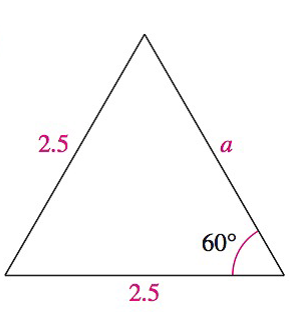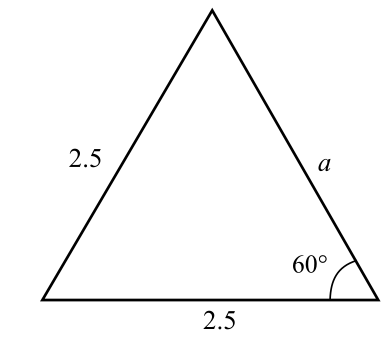Chapter 8.1, Problem 38E### Calculus: An Applied Approach (Min...

10th Edition
Ron Larson
ISBN: 9781305860919

#### Solutions

Chapter
Section### Calculus: An Applied Approach (Min...

10th Edition
Ron Larson
ISBN: 9781305860919
Textbook Problem
1 views

# Analyzing Triangles In Exercises 33-40, solve the triangle for the indicated side and/or angle.To determine

The value of side a in a triangle provided below.Explanation

Given Information:

The provided triangle is shown below,

In an isosceles triangle, two sides are equal and its two angles opposite to the sides are equal.

In an equilateral triangle, each angle is 60° and each side is equal.

From the observation of the triangle, the two sides have same length of 2.5 units

### Still sussing out bartleby?

Check out a sample textbook solution.

See a sample solution

#### The Solution to Your Study Problems

Bartleby provides explanations to thousands of textbook problems written by our experts, many with advanced degrees!

Get Started

#### Express the function in the form f g. 46. G(x)=x1+x3

Single Variable Calculus: Early Transcendentals, Volume I

#### True or False: is conservative.

Study Guide for Stewart's Multivariable Calculus, 8th

#### True or False: converges conditionally.

Study Guide for Stewart's Single Variable Calculus: Early Transcendentals, 8th